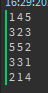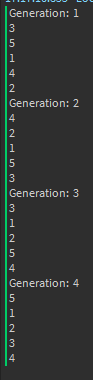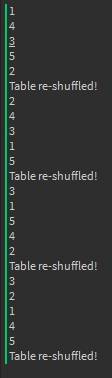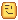# An efficient way to randomize a list of maps or items

If you’re making a round-based game that requires items or maps randomizing then I have a little tips for you.

Let’s start this by preparing the essentials. I will be making an example of randomizing maps every round.

``````local storage = game:GetService("ReplicatedStorage")
local mapsfolder = storage.Maps
local maps = mapsfolder:GetChildren()
``````

For the next step, have you ever thought or did something like this?

``````local function LoadMap(map)
map.Parent = workspace
end

while true do
local map = maps[math.random(1,#maps)] --  <== This?
end
``````

Well, I’m not saying it does not work, but it’s not an efficient way to do it, and there is a reason to it.
Let’s try `math.random(1,5)` and look at the output.See how many times the number 5 and 3 keep repeatedly appearing and the number 4 only appears twice?
This is why it’s not a good way to do it.

So what other methods are there that you can use?I will give you one method in billions of ways to do it.

Here’s my method

Let’s not use math.random to get an object from a table, but instead let’s shuffle the table!

``````math.randomseed(tick())

local nums = {1,2,3,4,5}
local gen = 0

while true do
Shuffle(nums) --This function will mix the nums' variables up randomly.
gen = gen + 1
print("Generation: ",gen)
for i=1,#nums do
print(nums[i])
end
wait(1)
end
``````

Pretty neat, right? Now let’s make ourselfs a shuffle function:

``````local function Shuffle(tabl)
for i=1,#tabl-1 do
local ran = math.random(i,#tabl)
tabl[i],tabl[ran] = tabl[ran],tabl[i]
end
end
``````

Now that we have everything that we need, let’s test it out and look at the output:Isn’t that just wonderful?
If we use this method to randomize maps for each round, all the maps will appear in order and no maps will be repeatedly appearing.

Let’s create a step variable to walk through the table and when it reaches the end of the table, the table will re-shuffle itself.

``````math.randomseed(tick())

local nums = {1,2,3,4,5}

local function Shuffle(tabl)
for i=1,#tabl-1 do
local ran = math.random(i,#tabl)
tabl[i],tabl[ran] = tabl[ran],tabl[i]
end
end

local step = 0

while true do
step = step + 1
print(nums[step])
if step == #nums then
Shuffle(nums)
print("Table re-shuffled!")
step = 0
end
wait(1)
end
``````And we’re finished!Have fun randomizing maps or items without getting too many duplicates.

45 Likes

I recommend posting the code directly to the forums rather than taking screenshots. It makes it easier for people to copy the code to try it out themselves. It also improves accessibility and searchability. Here is how to format code properly when posting it:

`````````lua
--Code goes here
/```
``````

Without the /

16 Likes

I have another way to shuffle an array which I think is easier

``````local toShuffle = {"one", "two", "three", "four"}

local function shuffleArray(arr)
local arrCopy = {unpack(arr)} -- # making copy of arr

for i = 1, #arr do
arr[i] = table.remove(arrCopy, math.random(#arrCopy))
end
return arr -- # arr has been shuffled, return back for convenience
end

print(table.concat(shuffleArray(toShuffle), ", "))
--> four, two, three, one
``````
12 Likes

Copying the entire table to shuffle it is a gigantic overhead though, making it problematic if not impossible at very large tables. The actual result appears to be equal to OP, since both are re-assigning each index to a random new value.

9 Likes

As long as both use tables with numeric indices, both methods work fine regardless of the value held in a key (assuming the tables are shallow). Or did you mean something else?

I believe @emojipasta is referring to the fact that I used `table.concat` to print the elements of the now shuffled table. But that is not the point of my function, and I do agree with the large tables issue.

1 Like

For your code sample, why do you keep maps in ReplicatedStorage? I’m curious to know what your rationale for this is.

In terms of the thread though, shuffling a table for pseudorandomisation is something I never bothered to implement in “random-based” map modes (normally I use an anchored cycle), rather thought of. I never really have a reason to shuffle tables. Cool tip, though.

I’m pretty sure that you could just do something like

``````for i = 1, 5 do
table.insert(nums, i)
end
``````

to easier insert numbers into a table without having to insert numbers into the table again, although the efficiency of something like this would become, unless I am wrong, a concern.

It’s possible they’re attempting to reduce download sizes when switching maps by simply re-parenting on the client rather than keeping it strictly server-side. Or just for the example.

1 Like

EDIT: This answer is incorrect. It can error out since `table.sort()` expects all values to consistently be higher or lower than others. Doing it randomly creates an inconsistency that causes an error. Instead, do a Fisher-Yates shuffle, like this one:

``````local rng = Random.new()

function shuffle<K, V>(from: {[K]: V}):{[K]: V}
for i = #from, 2, -1 do
local j = rng:NextInteger(1, i)
from[i], from[j] = from[j], from[i]
end
return from
end
``````

Hey, this is a bit old, but if anyone comes here for help: I think using `table.sort()` is much more easier, cleaner and efficient. A little bit hacky, but it works just as expected.

It’s easy, just use `sort` but completely ignore the values to compare, and return something random instead.

It should shuffle the table and randomize it. Here’s an example:

``````local tbl = {1,2,3,4,5,6}
table.sort(tbl, function()
return Random.new():NextNumber() < 0.5
end)

print(table.concat(tbl, ", ")) -- 6, 5, 1, 4, 2, 3
``````

Hope it helps!

51 Likes